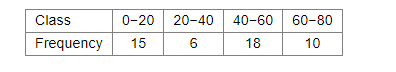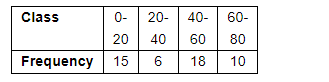# Find the mode of the following data:`
Question:

Find the mode of the following data:Solution:

We have to find the mode of the following distribution,The class (40-60) has the maximum frequency; therefore this is the modal class.

Lower limit of the modal class $x_{k}=40$

Width of the class interval h = 20

Frequency of the modal class fk = 18

Frequency of the class preceding the modal class fk−1 = 6

Frequency of the class succeeding the modal class fk+1 = 10

Now, we have the following formula to find the value of mode.

Mode $=x_{k}+h\left(\frac{f_{k}-f_{k-1}}{2 f_{k}-f_{k-1}-f_{k+1}}\right)$

$=40+20 \times\left(\frac{18-6}{2 \times 18-6-10}\right)$

$=40+2$

$=42$

Hence the value of mode is 42.